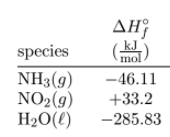# Problem: Determine the enthalpy of reaction for 4 NH3(g) + 7 O2(g) → 4 NO2(g) + 6 H2O(l) 1. −1397.6 kJ/mol rxn  2. +2034.4 kJ/mol rxn 3. −1899 kJ/mol rxn 4. −298.7 kJ/mol rxn 5. −1766 kJ/mol rxn

79% (9 ratings)
###### Problem Details

Determine the enthalpy of reaction for

4 NH3(g) + 7 O2(g) → 4 NO2(g) + 6 H2O(l)

1. −1397.6 kJ/mol rxn

2. +2034.4 kJ/mol rxn

3. −1899 kJ/mol rxn

4. −298.7 kJ/mol rxn

5. −1766 kJ/mol rxnWhat scientific concept do you need to know in order to solve this problem?

Our tutors have indicated that to solve this problem you will need to apply the Enthalpy of Formation concept. You can view video lessons to learn Enthalpy of Formation. Or if you need more Enthalpy of Formation practice, you can also practice Enthalpy of Formation practice problems.

What is the difficulty of this problem?

Our tutors rated the difficulty ofDetermine the enthalpy of reaction for 4 NH3(g) + 7 O2(g) →...as high difficulty.

How long does this problem take to solve?

Our expert Chemistry tutor, Dasha took 4 minutes and 41 seconds to solve this problem. You can follow their steps in the video explanation above.

What professor is this problem relevant for?

Based on our data, we think this problem is relevant for Professor Turnpenny's class at BU.